﻿ CT系统的参数标定及成像 CT System Parameter Calibration and Imaging

Vol.07 No.07(2018), Article ID:26142,15 pages
10.12677/AAM.2018.77108

CT System Parameter Calibration and Imaging

Hong Yang, Zhengtai Ma, Wenwen Ju

Minzu University of China, BeijingReceived: Jul. 6th, 2018; accepted: Jul. 23rd, 2018; published: Jul. 30th, 2018ABSTRACT

Aiming at the parameter calibration and imaging problem of CT system, this paper realizes the calibration of CT system parameters and the information of unknown medium through geometric analysis and graph reconstruction algorithm, and uses the accuracy and stability of parameter calibration to determine the better CT calibration template. We first analyze the relationship of the data and find the distance between the detector units, then establish a reasonable Cartesian coordinate system and use the geometric knowledge of the ellipse to calibrate the parameters of the CT system. In order to reconstruct the unknown image, we chose the filtered back projection algorithm (FBP) and the algebraic reconstruction algorithm (ART) to get information about the unknown medium and the absorption rate of 10 points. We use the original pattern and the reconstructed image of the calibration template to calculate the relative error of the distance between two points and the standard error of the CT value, so as to analyze the accuracy and stability of the calibration template. In order to improve it, based on the Caphan phantom, a new calibration template was established. After verification, the accuracy and stability of the calibration of the new template parameters have been improved.

Keywords:CT System, Parameter Calibration, Image Reconstruction, Filtered Back Projection Algorithm, Algebraic Reconstruction Algorithm

CT系统的参数标定及成像1. 问题的提出与分析

1.1. 问题的提出

CT (Computed Tomography)可以在不破坏样品的情况下，利用样品对射线能量的吸收特性获取样品内部的结构信息。本题中，我们考虑一种典型的二维CT：

1) 平行入射的X射线垂直于探测器平面，每个探测器单元看成一个接收点且等距排列。2) X射线的发射器和探测器相对位置保持不变，整个系统绕某固定的旋转中心逆时针旋转180次。3) 对每一个X射线方向，在具有512个等距单元的探测器上测量经位置固定不动的二维待检测介质吸收衰减后的射线能量，并经过增益等处理后得到180组接收信息。

CT系统安装时往往存在误差，为了保证成像质量，因此需要对安装好的CT系统进行参数标定，即借助于已知结构的样品(称为模板)标定CT系统的参数，并据此对未知结构的样品进行成像。

1) 在正方形托盘上放置两个均匀固体介质组成的标定模板，利用该模板的几何信息和接受信息确定CT系统旋转中心在正方形托盘中的位置、探测器单元之间的距离以及该CT系统使用的X射线的180个方向。

2) 基于(1)中得到的标定参数，利用上述CT系统得到的某未知介质的接收信息，确定该未知介质在正方形托盘中的位置、几何形状和吸收率等信息以及题中表3所给的10个位置处的吸收率。

3) 利用上述CT系统得到的另一未知介质的接收信息，确定该未知介质的相关信息10个位置处的吸收率。

4) 分析(1)中参数标定的精度和稳定性。在此基础上自行设计新模板、建立对应的标定模型，以改进标定精度和稳定性，并说明理由。

1.2. 问题的分析

1.2.1. 问题一：利用几何关系求得模板的参数信息

1.2.2. 问题二：利用图形重建算法确定未知介质信息

1.2.3. 问题三：利用图形重建算法确定未知介质信息

1.2.4. 问题四：分析精度和稳定性并改进标定模板

2. 模型假设

1) 假设CT系统平行入射的X射线光子波动不明显，忽略衍射现象。

2) 假设整个CT系统只有探测单元发出X射线，忽略X射线的光子能量发生电子对效应产生的X射线。

3) 假设题目所给的数据收集于正常工作的CT系统，且能反映实际情况。

4) 假设X射线源焦点和探测器单元为质点。

3. 符号说明

4. 模型的建立与求解

4.1. 问题一

4.1.1. 探测器单元距离的确定

180个探测方向经过均匀的小圆介质时的有接收信息的探测器个数N固定，即：

$N=29$

${d}_{\text{c}}=8\text{\hspace{0.17em}}\text{mm}$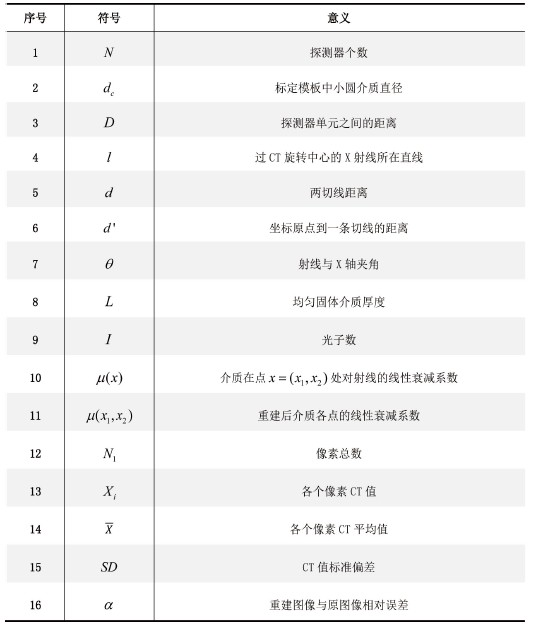Figure 1. Symbol description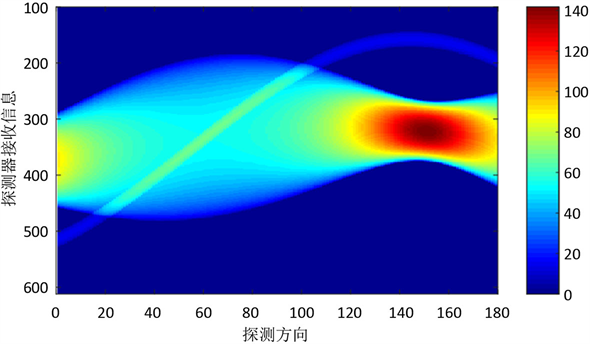Figure 2. Schematic diagram of receiving direction information

$\frac{D}{1}=\frac{{d}_{\text{c}}}{N}$ (1)

$D=0.2759\text{\hspace{0.17em}}\text{mm}$

4.1.2. 旋转中心和X射线180个方向的确定

$\left\{\begin{array}{l}d=\frac{2|\frac{{40}^{2}}{{y}_{0}}|}{\sqrt{\frac{{64}^{2}{x}_{0}^{2}}{{9}^{2}{y}_{0}^{2}}+1}}\\ \frac{{x}_{0}^{2}}{{15}^{2}}+\frac{{y}_{0}^{2}}{{40}^{2}}=1\end{array}$ (2)

$\theta =\mathrm{arctan}\left(\frac{64{x}_{0}}{9{y}_{0}}\right)$ (3)

$y=\frac{64{x}_{0}}{9{y}_{0}}x+\frac{{d}^{\prime }}{\mathrm{cos}\theta }$ (4)

1) 求旋转中心的确定

2) X射线180个方向的确定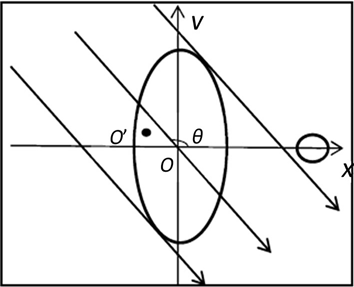Figure 3. Coordinate system

4.2. 问题二

CT图像重建算法有二维傅里叶变换法、反投影法、迭代法、拟合逼近法等，其中较常用的有反投影法中的滤波反投影算法(FBP)和代数重建算法(ART)  。基于问题一的模板，我们用这两种方法对图形进行重建，然后比较这两种算法的优劣，选择一种较优方法解决问题二。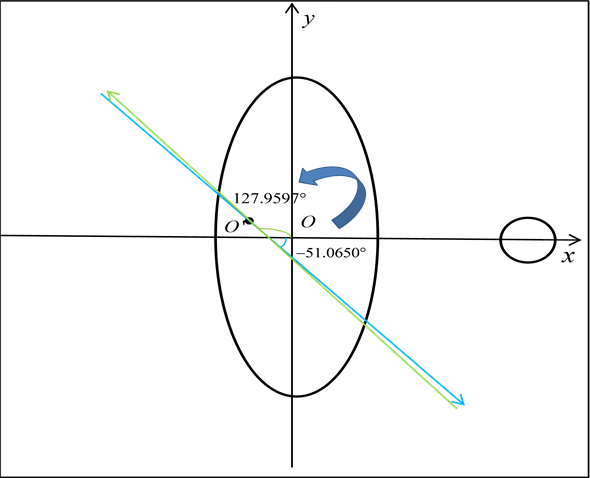Figure 4. CT system rotation

4.2.1. 平行束投影及其反演

$I={I}_{0}\mathrm{exp}\left(-\mu L\right)$ (5)

$I\left(L\right)={I}_{0}\mathrm{exp}\left(-{\int }_{L}\mu \left({x}_{1},{x}_{2}\right)\text{d}l\right)$ (6)

CT反演问题：利用CT系统的平行束射线探测未知介质，得到未与介质作用的光子数，进而重建介质各点的线性衰减系数 $\mu \left({x}_{1},{x}_{2}\right)$ 的值。因为线性衰减系数与介质的厚度和密度有线性关系，所以可以反映出未知介质的几何形状。

4.2.2. 滤波反投影算法(FPB)

1)中心切片定理

2)傅里叶变换法

$\mu \left(x\right)={\int }_{0}^{\text{2π}}{\int }_{0}^{+\infty }\overline{\mu }\left(\omega \varphi \right){\text{e}}^{i2\text{π}x\cdot \left(\omega \varphi \right)}\omega \text{d}\omega \text{d}\phi$ (7)

$\overline{\mu }\left(\omega \varphi \right)={\iint }_{{R}^{2}}\mu \left(x\right){\text{e}}^{-i2\text{π}x\cdot \left(\omega \varphi \right)}\text{d}x$ (8)

$\mu \left(x\right)={\int }_{0}^{n}{\left[{\int }_{-\infty }^{+\infty }\stackrel{˜}{p}\left(k,b\right)|w|W\left(\omega \right){\text{e}}^{-i2\text{π}rw}\text{d}w\right]}_{r=x\phi }\text{d}\varphi$ (9)

$\mu \left(x\right)={\int }_{0}^{\text{π}}{\left[{\int }_{-\infty }^{+\infty }\stackrel{˜}{p}\left(\omega ,\phi \right)|\omega |W\left(\omega \right){\text{e}}^{i2\text{π}r\omega }\text{d}\omega \right]}_{r=x\cdot \varphi }\text{d}\phi$ (10)

$\stackrel{˜}{p}\left(\omega ,\phi \right)={\int }_{-\infty }^{+\infty }p\left(r,\phi \right){\text{e}}^{-i2\text{π}r\omega }\text{d}r$ (11)

4.2.3. 代数重建算法(ART)

1) 对X赋值 $X={X}^{\left(0\right)}$

2) 沿逐条射线迭代

${X}^{\left(i\right)}={X}^{i-1}+{\lambda }^{\left(k\right)}\left(\frac{b}{‖{A}_{i}‖}-\frac{{A}_{i}}{‖{A}_{i}‖}{X}^{\left(i-1\right)}\right)\frac{{A}_{i}^{T}}{‖{A}_{i}‖},\text{\hspace{0.17em}}i=0,1,\cdots ,I$ (12)

3) 反复计算(2)式，直到满足一定的收敛条件，比如判断 ${‖A{X}^{\left(k\right)}-B‖}^{2}=\sum _{i=1}^{I}{\left({A}_{i}{X}^{\left(k\right)}-{b}_{i}\right)}^{2}$ 是否小于给定的阈值  。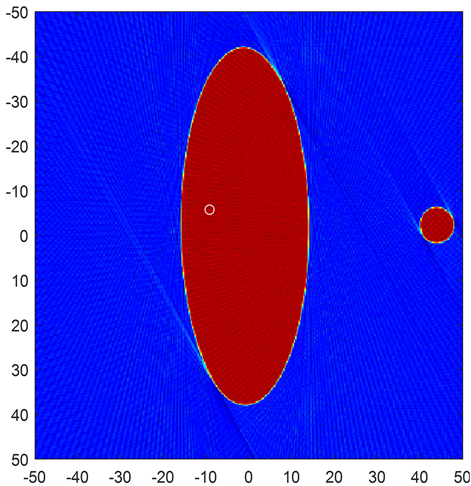Figure 5. CT image of FPB reconstruction calibration template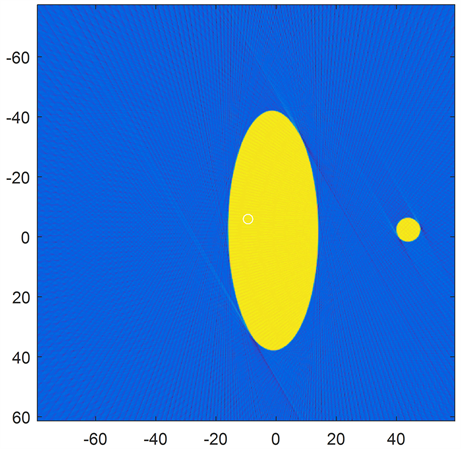Figure 6. CT image of calibration template by algebraic reconstruction algorithm

4.2.4. CT重建图像噪声分析

$SD=\sqrt{\frac{\sum _{i=1}^{N}{\left({X}_{i}-\overline{X}\right)}^{2}}{N-1}}$ (13)

4.2.5. 模型求解

4.3. 问题三Figure 7. CT value curve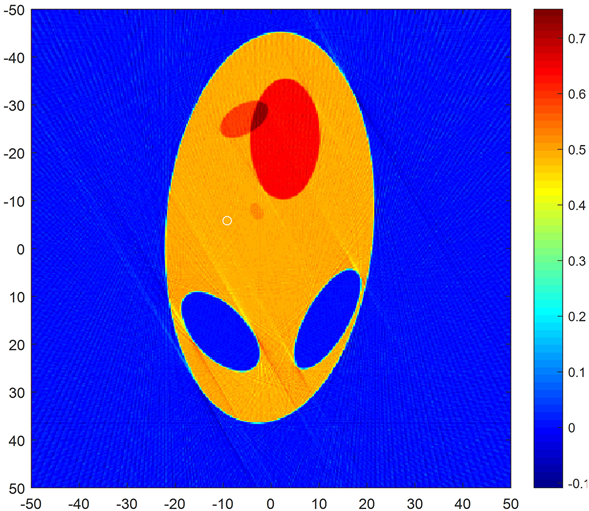Figure 8. Problem 2 reconstruction imageTable 3. Absorption rates at 10 locations in question 2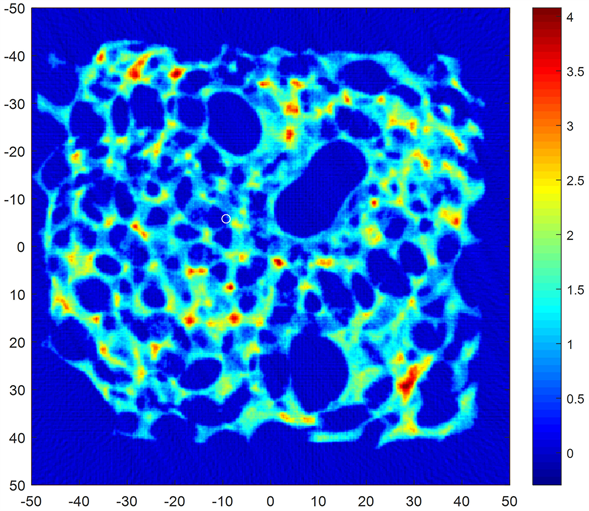Figure 9. Problem 3 reconstructed imageTable 4. Absorption rates at 10 locations in problem 3

4.4. 问题四

4.4.1. 问题一中参数标定的精度

$\alpha =\frac{|\Delta {x}^{\prime }-\Delta x|}{\Delta x}×100%$

4.4.2. 问题一中参数标定的稳定性

$SD=\sqrt{\frac{\sum _{i=1}^{N}{\left({X}_{i}-\overline{X}\right)}^{2}}{N-1}}$ (14)

4.4.3. 设定新的标定模板

$\Delta x=20$$\Delta {x}^{\prime }=19.9219$$\alpha =0.3906$$S{D}_{1}=0.0000$

5. 模型优缺点分析

5.1. 优点

1) 滤波反投影算法(FBP)：数学形式较为简单，应用广泛，容易达到预期效果，在锥角较小、扫描半径较大情况下能够取得较好的重建效果。

2) 代数重建算法(ART)：适合不完全投影数据的图像重建，当投影数据不完整时可以把缺失的数据看成是缺少了几个方程，这在某种程度上忽略了数据不全的问题。因而适用于不能获得完整投影数据的图像重建。且重建质量好，尤其是在投影数据较少的情况下。重建算法简单，适用于对不同格式的采样数据重建。缺点是计算量大，重建速度较慢。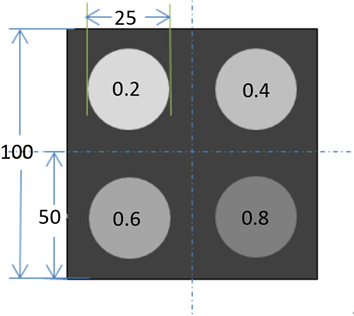Figure 10. New calibration templateTable 5. Comparison of the two calibration tables

5.2. 缺点

ART当投影数据不完整时可以把缺失的数据看成是缺少了几个方程。这在某种程度上忽略了数据不全的问题。因而适用于不能获得完整投影数据的图像重建。缺点是重建速度较慢。

CT System Parameter Calibration and Imaging[J]. 应用数学进展, 2018, 07(07): 903-917. https://doi.org/10.12677/AAM.2018.77108

1. 1. 骆岩红. CT图像重建滤波反投影算法中指数滤波器的研究[J]. 计算机科学, 2014, 41(S1): 220-223.

2. 2. 张朋, 张慧滔, 赵云松. X射线CT成像的数学模型及其有关问题[J]. 数学建模及其应用, 2012, 1(1): 1-12.

3. 3. 王洋, 夏顺仁, 汪元美. X射线CT模型及重建算法[J]. 计算机工程与应用, 2005(4): 220-222, 229.

xc =-5.7939; yc =-9.2427;

phantom = [zeros(100,180); phantom; zeros(100,180)];

imagesc(phantom)

figure

n = size(img,1);

[x,y]=meshgrid([-n/2:n/2]*0.2759);

imagesc(x(1,:)-10, y(:,1)-8, img)

hold on

plot(yc,xc,'ow')

axis image

clc;

clear all;

close all;

N = 256;

N2 = N^2;

theta = linspace(0,180,181);

theta = theta(1:180);

P_num =512;

delta = 1;

[W_ind,W_dat] = SystemMatrix(theta,N,P_num,delta);

F = zeros(N2,1);

lambda = 0.25;

c = 0;

irt_num = 5;

while(c

for j = 1:length(theta)

for i = 1:1:P_num

u = W_ind((j-1)*P_num + i,:);

v = W_dat((j-1)*P_num + i,:);

if any(u) == 0

continue;

end

w = zeros(1,N2);

ind = u > 0;

w(u(ind))=v(ind);

PP = w * F;

C = (P(i,j)-PP)/sum(w.^2) * w';

F = F + lambda * C;

end

end

F(F<0) = 0;

c = c+1;

end

F = reshape(F,N,N)';

figure(1);

imagesc(I);xlabel('(a)信息数据图像');

figure(2);